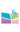﻿2022.2.24，李春霞 教授，首都师范大学，q-Difference two-dimensional Toda lattice equation, q-difference sine-Gordon equation and their integrability-中国矿业大学（北京）理学院网站2022.2.24，李春霞 教授，首都师范大学，q-Difference two-dimensional Toda lattice equation, q-difference sine-Gordon equation and their integrability
 题  目： q-Difference two-dimensional Toda lattice equation, q-difference sine-Gordon equation and their integrability 报告人： 李春霞 教授，首都师范大学 摘  要： In our previous work, we constructed quasi-Casoratian solutions to the noncommutative q-difference two-dimensional Toda lattice (q-2DTL) equation by Darboux transformation, which, in commutative setting, transforms to the existing Casoratian solutions to the bilinear q-2DTL equation obtained by Hirota's bilinear method. It is actually true that one can not only construct solutions to soliton equations but also solutions to their corresponding Backlund transformations by their Darboux transformations and binary Darboux transformations. To be more specific, eigenfunctions produced by iterating Darboux transformations and binary Darboux transformations for soliton equations give nothing but determinant solutions to their Backlund transformations, individually. This reveals the profound connections between Darboux transformations and Hirota's bilinear method. In this talk, we shall expound this point of view in the case of the q-2DTL equation. First, we derive a generalized Backlund transformation and Lax pair for the q-2DTL equation. And then we successfully construct the binary Darboux transformation for the q-2DTL equation, based on which, Grammian solutions expressed in terms of quantum integrals are established for both of the q-2DTL equation and its Backlund transformation. Finally, by imposing the 2-periodic reductions on the corresponding results of the q-2DTL equation, we derive a q-difference sine-Gordon equation, a modified q-difference sine-Gordon equation and obtain their corresponding solutions. 时  间： 2月24日 星期四 9:00—10:00 方  式： 腾讯会议会议 ID：435 795 374会议密码：0224 邀请人： 田凯 副教授